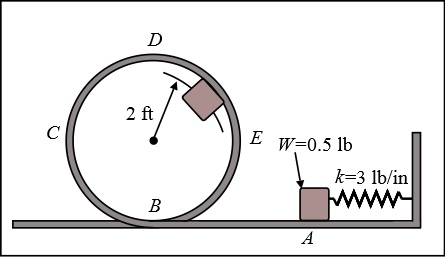# The 0.5-lb block is pushed against the spring at A and released from rest. Neglecting friction,...

## Question:

The 0.5-lb block is pushed against the spring at A and released from rest.

Neglecting friction, determine the smallest deflection of the spring for which the block will travel around the loop ABCDE and remain at all times in contact with the loop.## Energy Conservation:

The energy at every point for a particular system always remains equal as defined by the law of energy conservation. It means that the form of energy for a particular object can be changed, but its value remains the same.

## Answer and Explanation:

Given data:

• Weight of the block is: {eq}W = 0.5\;{\rm{lb}} {/eq}
• Spring constant is: {eq}k = 3\;{\rm{lb/in}} = 3\;{\rm{lb/in}} \times \dfrac{{12\;{\rm{lb/ft}}}}{{1\;{\rm{lb/in}}}} = 36\;{\rm{lb/ft}} {/eq}
• Radius of the loop is: {eq}r = 2\;{\rm{ft}} {/eq}

Expression for the weight of the block.

{eq}W = mg {/eq}

Here, the mass of the block is {eq}m {/eq} and the acceleration due to gravity is {eq}g = 32.2\;{\rm{ft/}}{{\rm{s}}^2}. {/eq}

Substitute the values in the above expression.

{eq}\begin{align*} 0.5\;{\rm{lb}} &= m\left( {32.2\;{\rm{ft/}}{{\rm{s}}^2}} \right)\\ m &= 0.015527\;{\rm{lb}} \cdot {{\rm{s}}^2}/{\rm{ft}} \end{align*} {/eq}

Equating the forces.

{eq}W = \dfrac{{m{v_D}^2}}{r} {/eq}

Here, the velocity of the block at D is {eq}{v_D}. {/eq}

Substitute the values in the above expression.

{eq}\begin{align*} m\left( {32.2\;{\rm{ft/}}{{\rm{s}}^2}} \right) &= \dfrac{{m{v_D}^2}}{{2\;{\rm{ft}}}}\\ {v_D}^2 &= 64.4\;{\rm{f}}{{\rm{t}}^2}/{{\rm{s}}^2} \end{align*} {/eq}

Expression for the initial spring energy.

{eq}S.{E_1} = \dfrac{1}{2}k{x^2} {/eq}

Here, the smallest deflection of the spring for which the block will travel around the loop ABCDE and remain at all times in contact with the loop is {eq}x. {/eq}

Substitute the values in the above expression.

{eq}\begin{align*} S.{E_1} &= \dfrac{1}{2}\left( {36\;{\rm{lb/ft}}} \right) \times {x^2}\\ S.{E_1} &= 18{x^2} \end{align*} {/eq}

Expression for the final potential energy.

{eq}P.{E_2} = mg\left( {2r} \right) {/eq}

Substitute the values in the above expression.

{eq}\begin{align*} P.{E_2} &= 0.5\;{\rm{lb}}\left( {2 \times 2\;{\rm{ft}}} \right)\\ P.{E_2} &= 2\;{\rm{lb}} \cdot {\rm{ft}} \end{align*} {/eq}

Expression for the final kinetic energy.

{eq}K.{E_2} = \dfrac{1}{2}m{\left( {{v_D}} \right)^2} {/eq}

Substitute the values in the above expression.

{eq}\begin{align*} K.{E_2} &= \dfrac{1}{2}\left( {0.015527\;{\rm{lb}} \cdot {{\rm{s}}^2}/{\rm{ft}}} \right) \times 64.4\;{\rm{f}}{{\rm{t}}^2}/{{\rm{s}}^2}\\ K.{E_2} &= 0.499969\;{\rm{lb}} \cdot {\rm{ft}} \end{align*} {/eq}

Consider the conservation of energy.

{eq}S.{E_1} + P.{E_1} + K.{E_1} = S.{E_2} + P.{E_2} + K.{E_2} {/eq}

Here, the initial potential energy of the block is {eq}P.{E_1} = 0 {/eq}, the initial kinetic energy of the block is {eq}K.{E_1} = 0 {/eq} and the final spring energy is {eq}S.{E_2} = 0. {/eq}

Substitute the values in the above expression.

{eq}\begin{align*} 18{x^2} + 0 + 0 &= 0 + 2 + 0.499969\\ {x^2} &= 0.027776\\ x &= 0.1666\;{\rm{ft}} \end{align*} {/eq}

Thus, the smallest deflection of the spring for which the block will travel around the loop ABCDE and remain at all times in contact with the loop is 0.1666 ft.